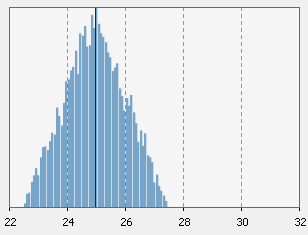AnyLogic

# triangularAVThis function is used for specifying distribution in the form like "roughly this, /- 20%".

Is equivalent to triangular( average * (1 - variability), average * (1 variability) ).

### triangularAV(double average, double variability)

Description
Generates a sample of the Triangular distribution with mode set to average.
Parameters
Name Type Description
average double the most likely x value
variability double the percent [0...1] of average representing the half of distribution range, where the generated sample falls
Result
Type Description
double the generated sample

### triangularAV(double average, double variability, Random r)

Description
Generates a sample of the Triangular distribution with mode set to average, and also uses the specified random number generator.
Parameters
Name Type Description
average double the most likely x value
variability double the percent [0...1] of average representing the half of distribution range, where the generated sample falls
r java.util.Random the random number generator
Result
Type Description
double the generated sample